# 纳米线基准模型的自洽薛定谔-泊松结果

2018年 10月 18日

### 求解薛定谔-泊松系统

#### 第 1 步

(1)

-\nabla\cdot(\epsilon\nabla V)=\rho

#### 第 2 步

(2)

V_e = qV

q 为载流粒子的电荷，其计算公式为

(3)

q=z_q~e

#### 第 4 步

{sum}

(4)

n_\mathrm{sum}
=\sum_i
{N_i}
~|\Psi_i|^2

(5)

N_i=g_i~\frac
{m_d} {\pi \hbar^2}k_B T~F_0\left(\frac{E_f-E_i}{k_B T}\right)~~~\mbox{1D}

(6)

N_i=g_i~\left(\frac

{2 m_d}
{\pi \hbar^2}
\right)^

{1/2} (k_B T)^{1/2}
~F_{-1/2}\left(\frac

{E_f-E_i}
{k_B T}
\right)~~~~\mbox

{1D axisymmetry or 2D}

(7)

N_i=g_i~\frac{2}{1+\exp\left(\frac{-E_f+E_i}{k_B T}
\right)}~~~~\mbox
{2D axisymmetry or 3D}

#### 第 5 步

{sum}
，我们重新计算空间电荷密度 \rho，并重新求解泊松方程，由此获得新的电势分布 V。新的空间电荷密度的简明算式为

(8)

\rho=q~n_\mathrm{sum}

(9)

\rho~=~q~n_\mathrm

{sum}
\exp\left(e^

{-\alpha}
~\frac{-q (V-V_\mathrm

{old}
)}

{k_B T}
\right)

{old}

(10)

\rho_\mathrm{new} = q~n_\mathrm{sum,old}

(11)

\rho_\mathrm
{new} = q~n_\mathrm{sum,new}

(12)

n_\mathrm

{sum,new}
= n_\mathrm

{sum,old}
~\exp\left(\frac{-q (V_\mathrm{new}-V_\mathrm

{old}
)}

{k_B T}
\right)

\alpha=0 时，得到 Eq. 9。此公式相当适用于高温条件，此时玻尔兹曼统计是一个很好的近似值。在较低温度下，将 \alpha 设为正数有利于加快收敛。

### 基准示例：纳米线模型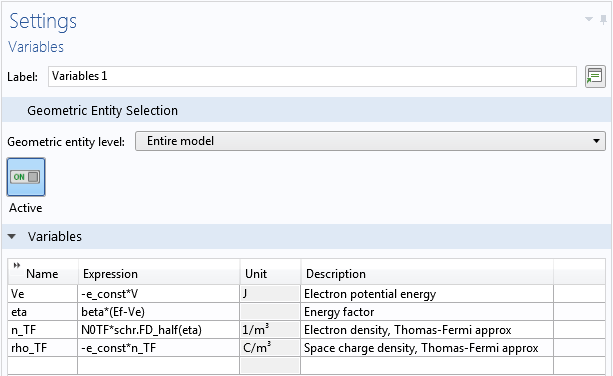• 如上文所述，角量子数 m被参数化，以便对其值进行扫描与求和，然后输入到薛定谔方程 物理场节点的设置 窗口中
• 回想关于计算超晶格带隙的博客文章，特征值尺度 λscale 与无量纲特征值 λ 相乘，得到特征能量 E_iE_i = λscale λ）
• 举例来说，如果 λscale 等于1 eV，特征值为 1.23，则特征能量等于 1.23 eV
• 如上文所述，在静电 接口中添加电势 边界条件，并设定纳米线的线壁的值
• 此外，添加了两个空间电荷密度 域条件，一个用于电离掺杂杂质，另一个用于 Thomas-Fermi 近似（在薛定谔-泊松研究 中应该禁用后一项）

### 创建薛定谔-泊松多物理场耦合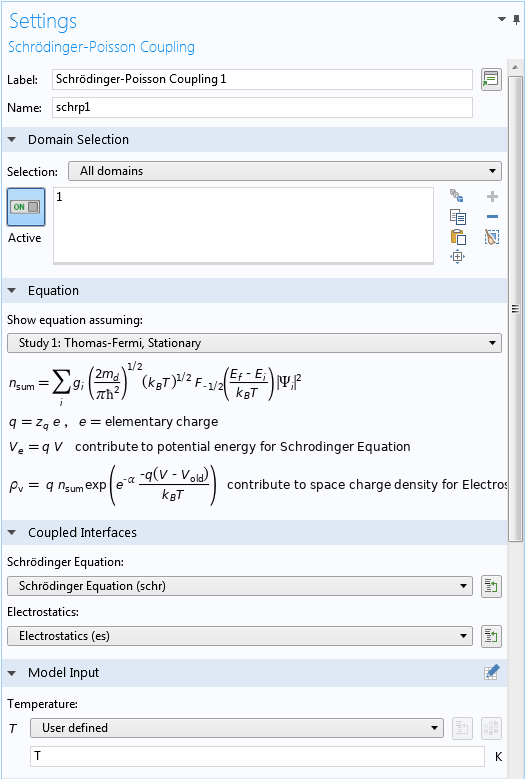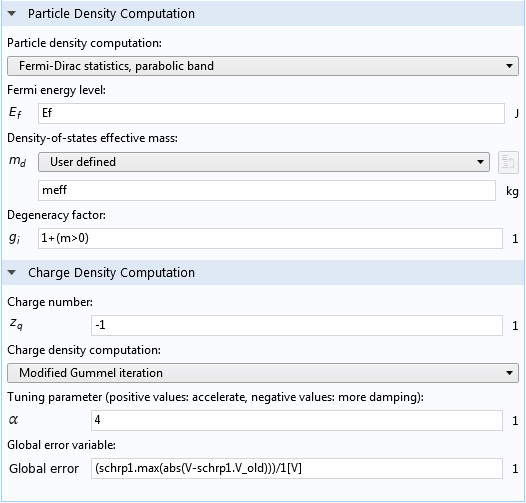### 建立薛定谔-泊松研究步骤

“研究 2”下的薛定谔-泊松 研究步骤自动在求解器序列中生成了自洽迭代。上文中介绍求解薛定谔-泊松系统的章节概述了迭代方案。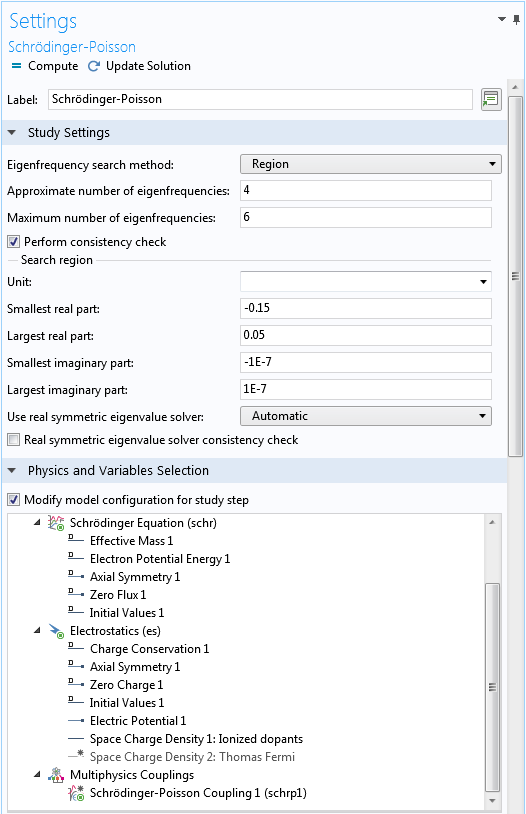### 检查自洽结果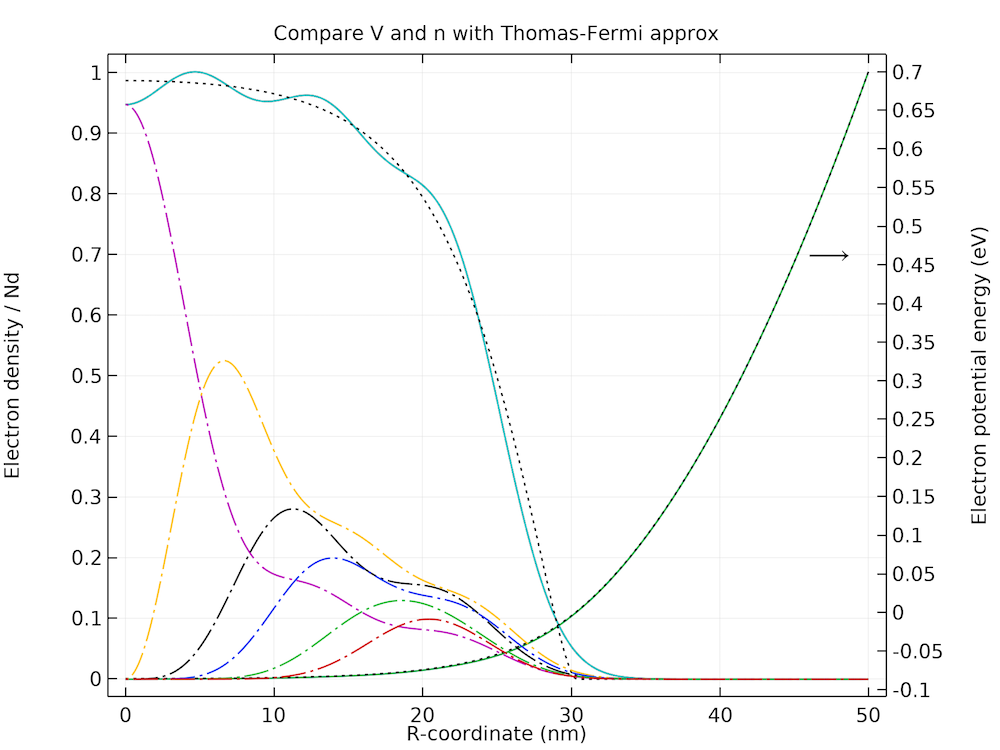### 参考文献

1. J.H. Luscombe, A.M. Bouchard, and M. Luban, “Electron confinement in quantum nanostructures: Self-consistent Poisson-Schrödinger theory,” Phys. Rev. B, vol. 46, no. 16, p. 10262, 1992.